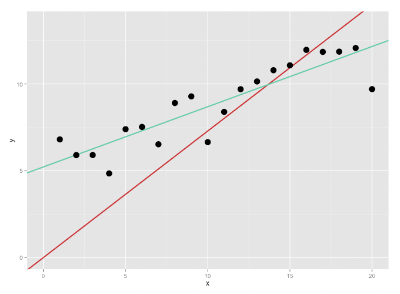## 2013-01-08

### Why we need the intercept ...

This is the first article in the series on non-hierarchical regression models. In this article we discuss the pitfalls when performing a regression without using the intercept term.

A common question is, if the intercept-term may be removed from a regression analysis in case it is not significant. Most of the time the answer to this question should be "No!" and I totally agree with that answer. But of course we would like to understand why!

We will use the following terminology:

1. A full model is a model containing the intercept and main effects: $y = b_0 + b_1\cdot x + \epsilon$
2. A no-intercept-model reduces the full model by its intercept: $y = b_1 \cdot x + \epsilon$
3. The intercept-only-model (often: baseline model) uses only the intercept to explain the data: $y = b_1 + \epsilon$

### Problems of no-intercept-models

1. $R^2$ is not useful any more.
2. The slope estimators might be biased.

### Explanation

The first commonly mentioned problem (see [1.]) is that the $R^2$-statistic is not useful anymore if the intercept is not included in a linear regression model. Usually $R^2$ is interpreted as the amount of variation that is explained by the model.

$$R^2 = \frac{Model SS}{Total SS} = 1 - \frac{Residual SS}{Total SS}$$

More precise: The full model is compared to a reduced model, which in this case is the intercept-only-model. So what do we do for an no-intercept-model? Well we will not compare it to the intercept-only-model. Both models are completely independent from each other so there is no sense in comparing. Instead most software packages (R, JMP, SAS, DX, SPSS, …) compare the model without intercept to a reference model that has lower order. That is a model with no intercept and no other effects.

$$\text{Noise-Model}: y = \epsilon$$

One might call it a noise-model. Of course this is no real model (it does not explain anything) and any comparison with it is not very useful. Actually there is no real reference model we could use to compare our no-intercept-model with. So there is no interpretable $R^2$ for models w/o intercept.

None the less statistics software will present an $R^2$ for no-intercept models. But as the interpretation of the $R^2$ is lost we cannot use it to evaluate the model quality. One can even show that the $R^2$ of a no-intercept model will usually be higher compared to the $R^2$ of a full model (see the mathematical details for that).

For the example presented in the graph below the $R^2s$ (calculated with R) are:

 $R^2$ Full Model 0.7846 No-Intercept-Model 0.9114

It is obvious that this is not a reasonable result. The red line is clearly not the better model!

The second problem that arises is that the least squares estimator for the slopes in a no-intercept model are biased (systematically shifted towards larger or smaller values).Usual (teal) and regression without intercept (red)

With removing the intercept from the model we impose a restriction so that the regression line goes through the origin (x=0;y=0). The graph shows what happens to the regression line. The blue line is the common regression line the red line is the no-intercept-regression-line. It is heavily pulled down because it has to go through $(0;0)$.

### Mathematical detailsR^2 does not work for no-intercept-modelsProof that LS-estimator is biased in no-intercept models

### When to use a no-intercept regression

Basically there is only one reason to perform a regression without using the intercept: Whenever your model is used to describe a process which is known to have a zero-intercept. Examples will be presented in the last article of this series.

So stay tuned!

### Literature

[1.] $R^2$-problem on CrossValidated: Link.
[2.] William Greene: Econometrics (Link; Biasedness of the OLS-estimator for omitted variables in Sections 4.3.2)